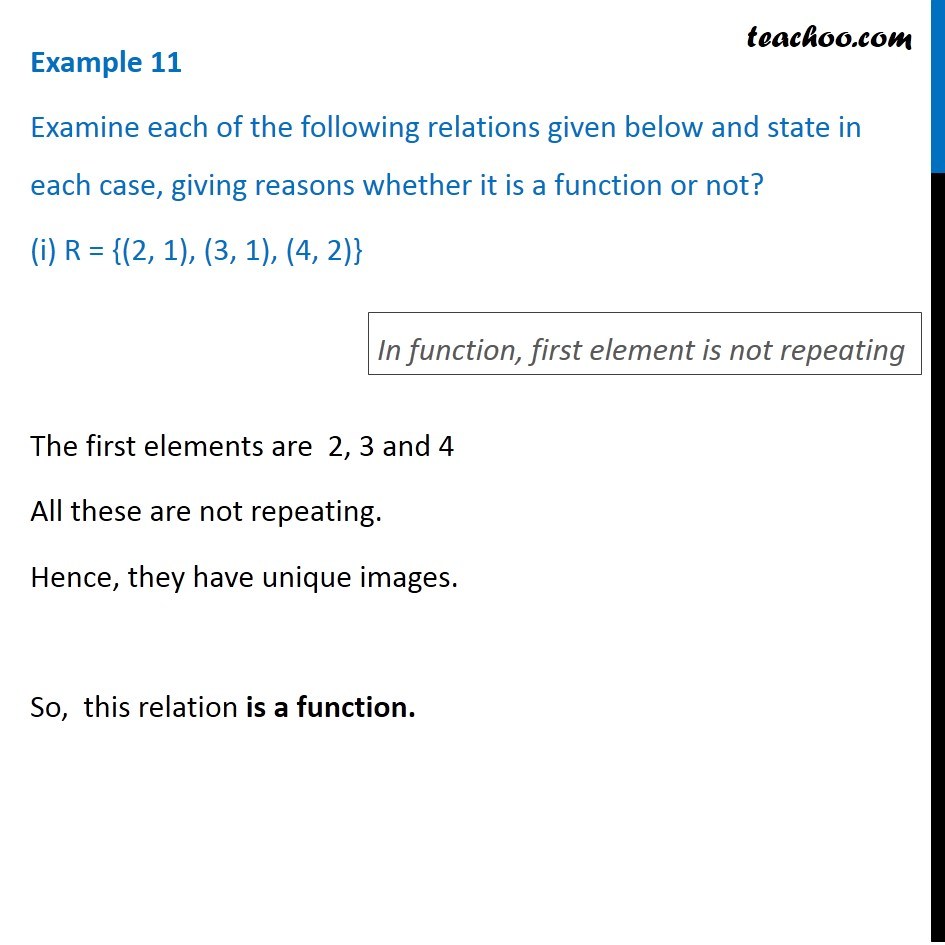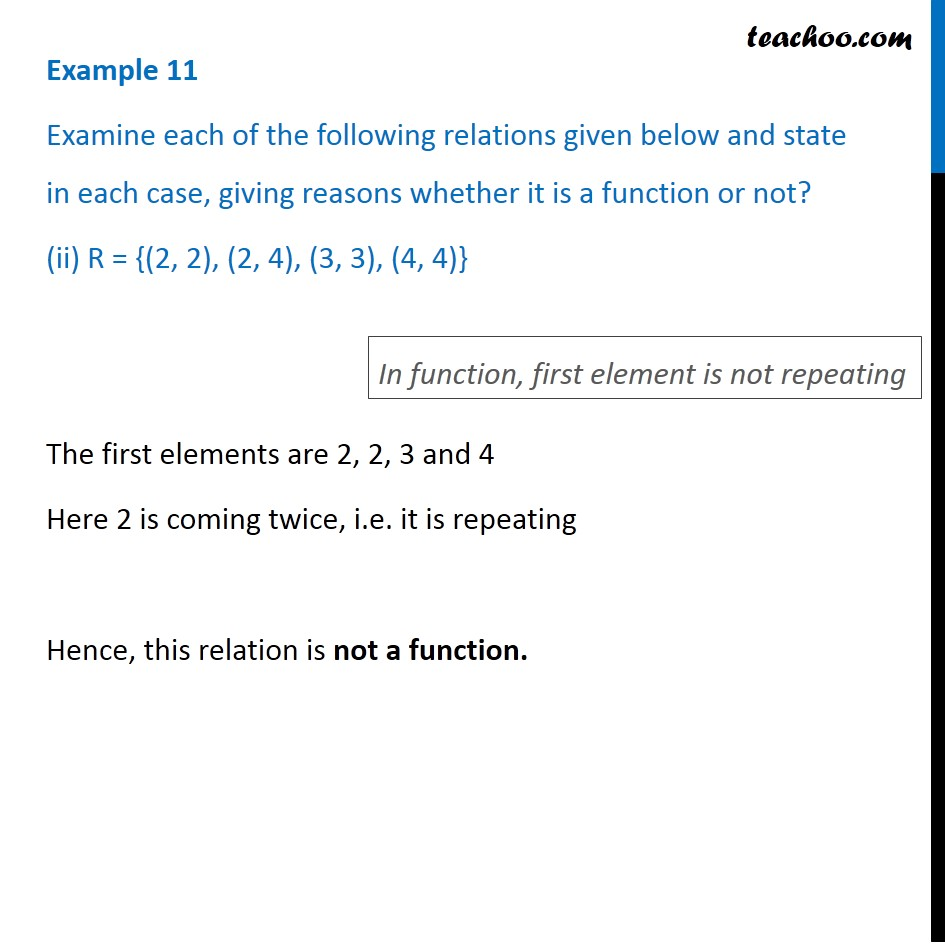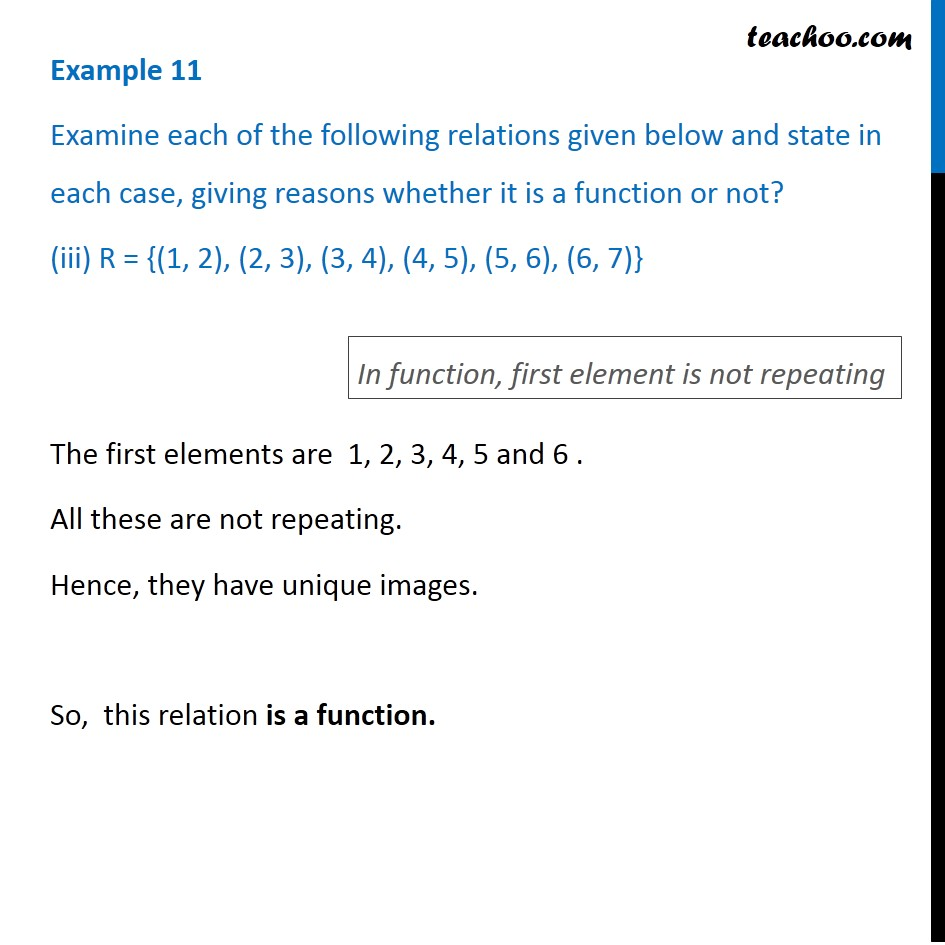Learn All Concepts of Chapter 2 Class 11 Relations and Function - FREE. Check - Relation and Function Class 11 - All Concepts1. Chapter 2 Class 11 Relations and Functions
2. Concept wise
3. Functions - Definition

Transcript

Example 11 Examine each of the following relations given below and state in each case, giving reasons whether it is a function or not? (i) R = {(2, 1), (3, 1), (4, 2)} The first elements are 2, 3 and 4 All these are not repeating. Hence, they have unique images. So, this relation is a function. In function, first element is not repeating Example 11 Examine each of the following relations given below and state in each case, giving reasons whether it is a function or not? (ii) R = {(2, 2), (2, 4), (3, 3), (4, 4)} The first elements are 2, 2, 3 and 4 Here 2 is coming twice, i.e. it is repeating Hence, this relation is not a function. In function, first element is not repeating Example 11 Examine each of the following relations given below and state in each case, giving reasons whether it is a function or not? (iii) R = {(1, 2), (2, 3), (3, 4), (4, 5), (5, 6), (6, 7)} The first elements are 1, 2, 3, 4, 5 and 6 . All these are not repeating. Hence, they have unique images. So, this relation is a function. In function, first element is not repeating

Functions - Definition